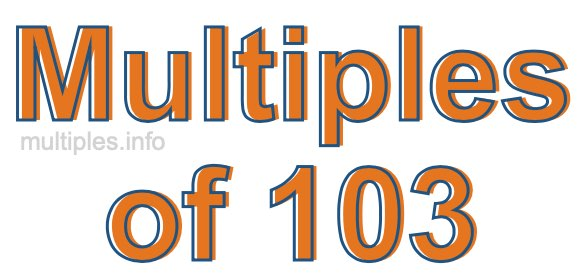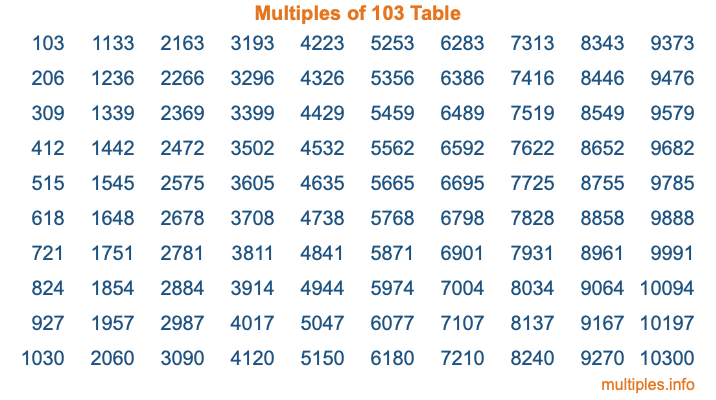Multiples of 103Welcome to the Multiples of 103 page. Here we will first teach you everything you will ever need to know about the multiples of 103, and then give you a study guide summary of everything we taught you to make sure you remember it all. Use this page to look up facts and learn information about the multiples of 103. This page will make you a multiples of one hundred three expert!

Definition of Multiples of 103
Multiples of 103 are all the numbers that when divided by 103 equal an integer. Each of the multiples of 103 are called a multiple. A multiple of 103 is created by multiplying 103 by an integer.

Therefore, to create a list of multiples of 103, you start with 1 multiplied by 103, then 2 multiplied by 103, then 3 multiplied by 103, and so on for as long as you want. Thus, the list of the first five multiples of 103 is 103, 206, 309, 412, and 515. To see a larger list of multiples of 103, see the printable image of Multiples of 103 further down on this page. We also have a category where you can choose any nth multiple of 103.

Multiples of 103 Checker
The Multiples of 103 Checker below checks to see if any number of your choice is a multiple of 103. In other words, it checks to see if there is any number (integer) that when multiplied by 103 will equal your number. To do that, we divide your number by 103. If the the quotient is an integer, then your number is a multiple of 103.

Is  a multiple of 103?

Least Common Multiple of 103 and ...
A Least Common Multiple (LCM) is the lowest multiple that two or more numbers have in common. This is also called the smallest common multiple or lowest common multiple and is useful to know when you are adding our subtracting fractions. Enter one or more numbers below (103 is already entered) to find the LCM.

Check out our LCM Calculator if you need more details about the Least Common Multiple or if you need the LCM for different numbers for adding and subtraction fractions.

nth Multiple of 103
As we stated above, 103 is the first multiple of 103, 206 is the second multiple of 103, 309 is the third multiple of 103, and so on. Enter a number below to find the nth multiple of 103.

th multiple of 103

Multiples of 103 vs Factors of 103
103 is a multiple of 103 and a factor of 103, but that is where the similarities end. All postive multiples of 103 are 103 or greater than 103. All positive factors of 103 are 103 or less than 103.

Below is the beginning list of multiples of 103 and the factors of 103 so you can compare:

Multiples of 103: 103, 206, 309, 412, 515, etc.

Factors of 103: 1, 103

As you can see, the multiples of 103 are all the numbers that you can divide by 103 to get a whole number. The factors of 103, on the other hand, are all the whole numbers that you can multiply by another whole number to get 103.

It's also interesting to note that if a number (x) is a factor of 103, then 103 will also be a multiple of that number (x).

Multiples of 103 vs Divisors of 103
The divisors of 103 are all the integers that 103 can be divided by evenly. Below is a list of the divisors of 103.

Divisors of 103: 1, 103

The interesting thing to note here is that if you take any multiple of 103 and divide it by a divisor of 103, you will see that the quotient is an integer.

Multiples of 103 Table
Below is an image of the first 100 multiples of 103 in a table. The table is in chronological order, column by column. The first column has the first ten multiples of 103, the second column has the next ten multiples of 103, and so on.The Multiples of 103 Table is also referred to as the 103 Times Table or Times Table of 103. You are welcome to print out our table for your studies.

Negative Multiples of 103
Although not often discussed or needed in math, it is worth mentioning that you can make a list of negative multiples of 103 by multiplying 103 by -1, then by -2, then by -3, and so on, to get the following list of negative multiples of 103:

-103, -206, -309, -412, -515, etc.

Multiples of 103 Summary
Below is a summary of important Multiples of 103 facts that we have discussed on this page. To retain the knowledge on this page, we recommend that you read through the summary and explain to yourself or a study partner why they hold true.

There are an infinite number of multiples of 103.

A multiple of 103 divided by 103 will equal a whole number.

103 divided by a factor of 103 equals a divisor of 103.

The nth multiple of 103 is n times 103.

The largest factor of 103 is equal to the first positive multiple of 103.

103 is a multiple of every factor of 103.

103 is a multiple of 103.

A multiple of 103 divided by a divisor of 103 equals an integer.

103 divided by a divisor of 103 equals a factor of 103.

Any integer times 103 will equal a multiple of 103.

Multiples of a Number
Here you can get the multiples of another number, all with the same attention to detail as we did for multiples of 103 on this page.

Multiples of
Multiples of 104
Did you find our page about multiples of one hundred three educational? Do you want more knowledge? Check out the multiples of the next number on our list!